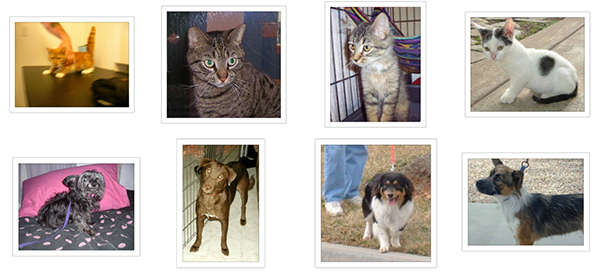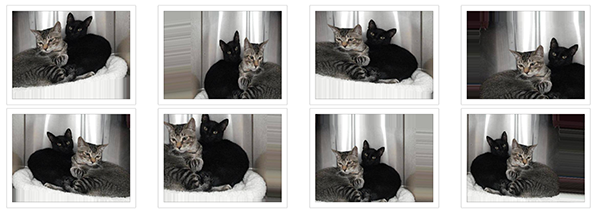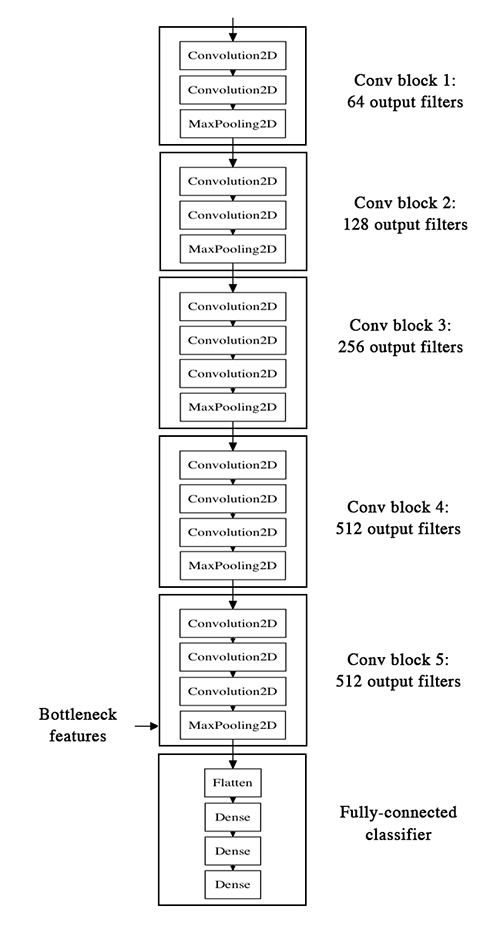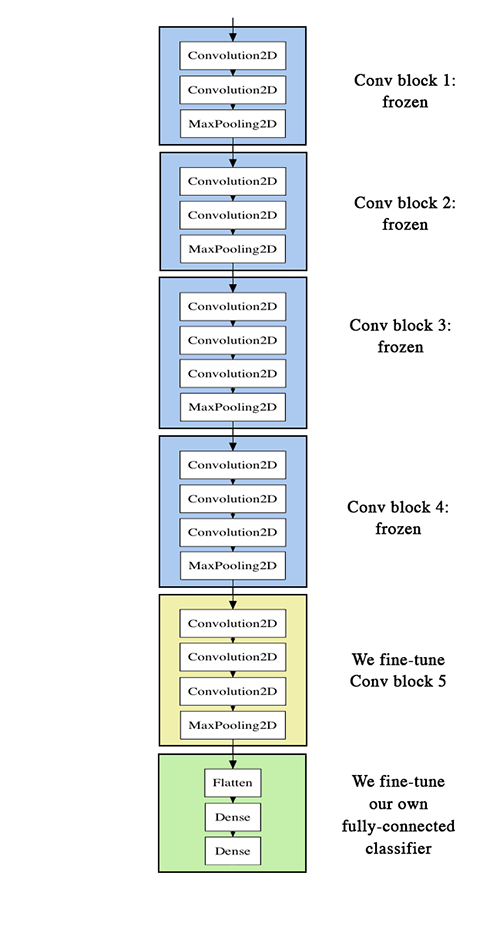# Building powerful image classification models using very little data

In this tutorial, we will present a few simple yet effective methods that you can use to build a powerful image classifier, using only very few training examples --just a few hundred or thousand pictures from each class you want to be able to recognize.

We will go over the following options:

• training a small network from scratch (as a baseline)
• using the bottleneck features of a pre-trained network
• fine-tuning the top layers of a pre-trained network

This will lead us to cover the following Keras features:

• `fit_generator` for training Keras a model using Python data generators
• `ImageDataGenerator` for real-time data augmentation
• layer freezing and model fine-tuning
• ...and more.

Note: all code examples have been updated to the Keras 2.0 API on March 14, 2017. You will need Keras version 2.0.0 or higher to run them.

## Our setup: only 2000 training examples (1000 per class)

We will start from the following setup:

• a machine with Keras, SciPy, PIL installed. If you have a NVIDIA GPU that you can use (and cuDNN installed), that's great, but since we are working with few images that isn't strictly necessary.
• a training data directory and validation data directory containing one subdirectory per image class, filled with .png or .jpg images:
```data/
train/
dogs/
dog001.jpg
dog002.jpg
...
cats/
cat001.jpg
cat002.jpg
...
validation/
dogs/
dog001.jpg
dog002.jpg
...
cats/
cat001.jpg
cat002.jpg
...
```

To acquire a few hundreds or thousands of training images belonging to the classes you are interested in, one possibility would be to use the Flickr API to download pictures matching a given tag, under a friendly license.

In our examples we will use two sets of pictures, which we got from Kaggle: 1000 cats and 1000 dogs (although the original dataset had 12,500 cats and 12,500 dogs, we just took the first 1000 images for each class). We also use 400 additional samples from each class as validation data, to evaluate our models.

That is very few examples to learn from, for a classification problem that is far from simple. So this is a challenging machine learning problem, but it is also a realistic one: in a lot of real-world use cases, even small-scale data collection can be extremely expensive or sometimes near-impossible (e.g. in medical imaging). Being able to make the most out of very little data is a key skill of a competent data scientist.How difficult is this problem? When Kaggle started the cats vs. dogs competition (with 25,000 training images in total), a bit over two years ago, it came with the following statement:

"In an informal poll conducted many years ago, computer vision experts posited that a classifier with better than 60% accuracy would be difficult without a major advance in the state of the art. For reference, a 60% classifier improves the guessing probability of a 12-image HIP from 1/4096 to 1/459. The current literature suggests machine classifiers can score above 80% accuracy on this task [ref]."

In the resulting competition, top entrants were able to score over 98% accuracy by using modern deep learning techniques. In our case, because we restrict ourselves to only 8% of the dataset, the problem is much harder.

## On the relevance of deep learning for small-data problems

A message that I hear often is that "deep learning is only relevant when you have a huge amount of data". While not entirely incorrect, this is somewhat misleading. Certainly, deep learning requires the ability to learn features automatically from the data, which is generally only possible when lots of training data is available --especially for problems where the input samples are very high-dimensional, like images. However, convolutional neural networks --a pillar algorithm of deep learning-- are by design one of the best models available for most "perceptual" problems (such as image classification), even with very little data to learn from. Training a convnet from scratch on a small image dataset will still yield reasonable results, without the need for any custom feature engineering. Convnets are just plain good. They are the right tool for the job.

But what's more, deep learning models are by nature highly repurposable: you can take, say, an image classification or speech-to-text model trained on a large-scale dataset then reuse it on a significantly different problem with only minor changes, as we will see in this post. Specifically in the case of computer vision, many pre-trained models (usually trained on the ImageNet dataset) are now publicly available for download and can be used to bootstrap powerful vision models out of very little data.

## Data pre-processing and data augmentation

In order to make the most of our few training examples, we will "augment" them via a number of random transformations, so that our model would never see twice the exact same picture. This helps prevent overfitting and helps the model generalize better.

In Keras this can be done via the `keras.preprocessing.image.ImageDataGenerator` class. This class allows you to:

• configure random transformations and normalization operations to be done on your image data during training
• instantiate generators of augmented image batches (and their labels) via `.flow(data, labels)` or `.flow_from_directory(directory)`. These generators can then be used with the Keras model methods that accept data generators as inputs, `fit_generator`, `evaluate_generator` and `predict_generator`.

Let's look at an example right away:

```from keras.preprocessing.image import ImageDataGenerator

datagen = ImageDataGenerator(
rotation_range=40,
width_shift_range=0.2,
height_shift_range=0.2,
rescale=1./255,
shear_range=0.2,
zoom_range=0.2,
horizontal_flip=True,
fill_mode='nearest')
```

These are just a few of the options available (for more, see the documentation). Let's quickly go over what we just wrote:

• `rotation_range` is a value in degrees (0-180), a range within which to randomly rotate pictures
• `width_shift` and `height_shift` are ranges (as a fraction of total width or height) within which to randomly translate pictures vertically or horizontally
• `rescale` is a value by which we will multiply the data before any other processing. Our original images consist in RGB coefficients in the 0-255, but such values would be too high for our models to process (given a typical learning rate), so we target values between 0 and 1 instead by scaling with a 1/255. factor.
• `shear_range` is for randomly applying shearing transformations
• `zoom_range` is for randomly zooming inside pictures
• `horizontal_flip` is for randomly flipping half of the images horizontally --relevant when there are no assumptions of horizontal assymetry (e.g. real-world pictures).
• `fill_mode` is the strategy used for filling in newly created pixels, which can appear after a rotation or a width/height shift.

Now let's start generating some pictures using this tool and save them to a temporary directory, so we can get a feel for what our augmentation strategy is doing --we disable rescaling in this case to keep the images displayable:

```from keras.preprocessing.image import ImageDataGenerator, array_to_img, img_to_array, load_img

datagen = ImageDataGenerator(
rotation_range=40,
width_shift_range=0.2,
height_shift_range=0.2,
shear_range=0.2,
zoom_range=0.2,
horizontal_flip=True,
fill_mode='nearest')

img = load_img('data/train/cats/cat.0.jpg')  # this is a PIL image
x = img_to_array(img)  # this is a Numpy array with shape (3, 150, 150)
x = x.reshape((1,) + x.shape)  # this is a Numpy array with shape (1, 3, 150, 150)

# the .flow() command below generates batches of randomly transformed images
# and saves the results to the `preview/` directory
i = 0
for batch in datagen.flow(x, batch_size=1,
save_to_dir='preview', save_prefix='cat', save_format='jpeg'):
i += 1
if i > 20:
break  # otherwise the generator would loop indefinitely
```

Here's what we get --this is what our data augmentation strategy looks like.## Training a small convnet from scratch: 80% accuracy in 40 lines of code

The right tool for an image classification job is a convnet, so let's try to train one on our data, as an initial baseline. Since we only have few examples, our number one concern should be overfitting. Overfitting happens when a model exposed to too few examples learns patterns that do not generalize to new data, i.e. when the model starts using irrelevant features for making predictions. For instance, if you, as a human, only see three images of people who are lumberjacks, and three, images of people who are sailors, and among them only one lumberjack wears a cap, you might start thinking that wearing a cap is a sign of being a lumberjack as opposed to a sailor. You would then make a pretty lousy lumberjack/sailor classifier.

Data augmentation is one way to fight overfitting, but it isn't enough since our augmented samples are still highly correlated. Your main focus for fighting overfitting should be the entropic capacity of your model --how much information your model is allowed to store. A model that can store a lot of information has the potential to be more accurate by leveraging more features, but it is also more at risk to start storing irrelevant features. Meanwhile, a model that can only store a few features will have to focus on the most significant features found in the data, and these are more likely to be truly relevant and to generalize better.

There are different ways to modulate entropic capacity. The main one is the choice of the number of parameters in your model, i.e. the number of layers and the size of each layer. Another way is the use of weight regularization, such as L1 or L2 regularization, which consists in forcing model weights to taker smaller values.

In our case we will use a very small convnet with few layers and few filters per layer, alongside data augmentation and dropout. Dropout also helps reduce overfitting, by preventing a layer from seeing twice the exact same pattern, thus acting in a way analoguous to data augmentation (you could say that both dropout and data augmentation tend to disrupt random correlations occuring in your data).

The code snippet below is our first model, a simple stack of 3 convolution layers with a ReLU activation and followed by max-pooling layers. This is very similar to the architectures that Yann LeCun advocated in the 1990s for image classification (with the exception of ReLU).

The full code for this experiment can be found here.

```from keras.models import Sequential
from keras.layers import Conv2D, MaxPooling2D
from keras.layers import Activation, Dropout, Flatten, Dense

model = Sequential()
model.add(Conv2D(32, (3, 3), input_shape=(3, 150, 150)))

# the model so far outputs 3D feature maps (height, width, features)
```

On top of it we stick two fully-connected layers. We end the model with a single unit and a sigmoid activation, which is perfect for a binary classification. To go with it we will also use the `binary_crossentropy` loss to train our model.

```model.add(Flatten())  # this converts our 3D feature maps to 1D feature vectors

model.compile(loss='binary_crossentropy',
optimizer='rmsprop',
metrics=['accuracy'])
```

Let's prepare our data. We will use `.flow_from_directory()` to generate batches of image data (and their labels) directly from our jpgs in their respective folders.

```batch_size = 16

# this is the augmentation configuration we will use for training
train_datagen = ImageDataGenerator(
rescale=1./255,
shear_range=0.2,
zoom_range=0.2,
horizontal_flip=True)

# this is the augmentation configuration we will use for testing:
# only rescaling
test_datagen = ImageDataGenerator(rescale=1./255)

# this is a generator that will read pictures found in
# subfolers of 'data/train', and indefinitely generate
# batches of augmented image data
train_generator = train_datagen.flow_from_directory(
'data/train',  # this is the target directory
target_size=(150, 150),  # all images will be resized to 150x150
batch_size=batch_size,
class_mode='binary')  # since we use binary_crossentropy loss, we need binary labels

# this is a similar generator, for validation data
validation_generator = test_datagen.flow_from_directory(
'data/validation',
target_size=(150, 150),
batch_size=batch_size,
class_mode='binary')
```

We can now use these generators to train our model. Each epoch takes 20-30s on GPU and 300-400s on CPU. So it's definitely viable to run this model on CPU if you aren't in a hurry.

```model.fit_generator(
train_generator,
steps_per_epoch=2000 // batch_size,
epochs=50,
validation_data=validation_generator,
validation_steps=800 // batch_size)
model.save_weights('first_try.h5')  # always save your weights after training or during training
```

This approach gets us to a validation accuracy of 0.79-0.81 after 50 epochs (a number that was picked arbitrarily --because the model is small and uses aggressive dropout, it does not seem to be overfitting too much by that point). So at the time the Kaggle competition was launched, we would be already be "state of the art" --with 8% of the data, and no effort to optimize our architecture or hyperparameters. In fact, in the Kaggle competition, this model would have scored in the top 100 (out of 215 entrants). I guess that at least 115 entrants weren't using deep learning ;)

Note that the variance of the validation accuracy is fairly high, both because accuracy is a high-variance metric and because we only use 800 validation samples. A good validation strategy in such cases would be to do k-fold cross-validation, but this would require training k models for every evaluation round.

## Using the bottleneck features of a pre-trained network: 90% accuracy in a minute

A more refined approach would be to leverage a network pre-trained on a large dataset. Such a network would have already learned features that are useful for most computer vision problems, and leveraging such features would allow us to reach a better accuracy than any method that would only rely on the available data.

We will use the VGG16 architecture, pre-trained on the ImageNet dataset --a model previously featured on this blog. Because the ImageNet dataset contains several "cat" classes (persian cat, siamese cat...) and many "dog" classes among its total of 1000 classes, this model will already have learned features that are relevant to our classification problem. In fact, it is possible that merely recording the softmax predictions of the model over our data rather than the bottleneck features would be enough to solve our dogs vs. cats classification problem extremely well. However, the method we present here is more likely to generalize well to a broader range of problems, including problems featuring classes absent from ImageNet.

Here's what the VGG16 architecture looks like:Our strategy will be as follow: we will only instantiate the convolutional part of the model, everything up to the fully-connected layers. We will then run this model on our training and validation data once, recording the output (the "bottleneck features" from th VGG16 model: the last activation maps before the fully-connected layers) in two numpy arrays. Then we will train a small fully-connected model on top of the stored features.

The reason why we are storing the features offline rather than adding our fully-connected model directly on top of a frozen convolutional base and running the whole thing, is computational effiency. Running VGG16 is expensive, especially if you're working on CPU, and we want to only do it once. Note that this prevents us from using data augmentation.

You can find the full code for this experiment here. You can get the weights file from Github. We won't review how the model is built and loaded --this is covered in multiple Keras examples already. But let's take a look at how we record the bottleneck features using image data generators:

```batch_size = 16

generator = datagen.flow_from_directory(
'data/train',
target_size=(150, 150),
batch_size=batch_size,
class_mode=None,  # this means our generator will only yield batches of data, no labels
shuffle=False)  # our data will be in order, so all first 1000 images will be cats, then 1000 dogs
# the predict_generator method returns the output of a model, given
# a generator that yields batches of numpy data
bottleneck_features_train = model.predict_generator(generator, 2000)
# save the output as a Numpy array
np.save(open('bottleneck_features_train.npy', 'w'), bottleneck_features_train)

generator = datagen.flow_from_directory(
'data/validation',
target_size=(150, 150),
batch_size=batch_size,
class_mode=None,
shuffle=False)
bottleneck_features_validation = model.predict_generator(generator, 800)
np.save(open('bottleneck_features_validation.npy', 'w'), bottleneck_features_validation)
```

We can then load our saved data and train a small fully-connected model:

```train_data = np.load(open('bottleneck_features_train.npy'))
# the features were saved in order, so recreating the labels is easy
train_labels = np.array( * 1000 +  * 1000)

validation_labels = np.array( * 400 +  * 400)

model = Sequential()

model.compile(optimizer='rmsprop',
loss='binary_crossentropy',
metrics=['accuracy'])

model.fit(train_data, train_labels,
epochs=50,
batch_size=batch_size,
validation_data=(validation_data, validation_labels))
model.save_weights('bottleneck_fc_model.h5')
```

Thanks to its small size, this model trains very quickly even on CPU (1s per epoch):

```Train on 2000 samples, validate on 800 samples
Epoch 1/50
2000/2000 [==============================] - 1s - loss: 0.8932 - acc: 0.7345 - val_loss: 0.2664 - val_acc: 0.8862
Epoch 2/50
2000/2000 [==============================] - 1s - loss: 0.3556 - acc: 0.8460 - val_loss: 0.4704 - val_acc: 0.7725
...
Epoch 47/50
2000/2000 [==============================] - 1s - loss: 0.0063 - acc: 0.9990 - val_loss: 0.8230 - val_acc: 0.9125
Epoch 48/50
2000/2000 [==============================] - 1s - loss: 0.0144 - acc: 0.9960 - val_loss: 0.8204 - val_acc: 0.9075
Epoch 49/50
2000/2000 [==============================] - 1s - loss: 0.0102 - acc: 0.9960 - val_loss: 0.8334 - val_acc: 0.9038
Epoch 50/50
2000/2000 [==============================] - 1s - loss: 0.0040 - acc: 0.9985 - val_loss: 0.8556 - val_acc: 0.9075
```

We reach a validation accuracy of 0.90-0.91: not bad at all. This is definitely partly due to the fact that the base model was trained on a dataset that already featured dogs and cats (among hundreds of other classes).

## Fine-tuning the top layers of a a pre-trained network

To further improve our previous result, we can try to "fine-tune" the last convolutional block of the VGG16 model alongside the top-level classifier. Fine-tuning consist in starting from a trained network, then re-training it on a new dataset using very small weight updates. In our case, this can be done in 3 steps:

• instantiate the convolutional base of VGG16 and load its weights
• add our previously defined fully-connected model on top, and load its weights
• freeze the layers of the VGG16 model up to the last convolutional blockNote that:

• in order to perform fine-tuning, all layers should start with properly trained weights: for instance you should not slap a randomly initialized fully-connected network on top of a pre-trained convolutional base. This is because the large gradient updates triggered by the randomly initialized weights would wreck the learned weights in the convolutional base. In our case this is why we first train the top-level classifier, and only then start fine-tuning convolutional weights alongside it.
• we choose to only fine-tune the last convolutional block rather than the entire network in order to prevent overfitting, since the entire network would have a very large entropic capacity and thus a strong tendency to overfit. The features learned by low-level convolutional blocks are more general, less abstract than those found higher-up, so it is sensible to keep the first few blocks fixed (more general features) and only fine-tune the last one (more specialized features).
• fine-tuning should be done with a very slow learning rate, and typically with the SGD optimizer rather than an adaptative learning rate optimizer such as RMSProp. This is to make sure that the magnitude of the updates stays very small, so as not to wreck the previously learned features.

You can find the full code for this experiment here.

After instantiating the VGG base and loading its weights, we add our previously trained fully-connected classifier on top:

```# build a classifier model to put on top of the convolutional model
top_model = Sequential()

# classifier, including the top classifier,
# in order to successfully do fine-tuning

# add the model on top of the convolutional base
```

We then proceed to freeze all convolutional layers up to the last convolutional block:

```# set the first 25 layers (up to the last conv block)
# to non-trainable (weights will not be updated)
for layer in model.layers[:25]:
layer.trainable = False

# compile the model with a SGD/momentum optimizer
# and a very slow learning rate.
model.compile(loss='binary_crossentropy',
optimizer=optimizers.SGD(lr=1e-4, momentum=0.9),
metrics=['accuracy'])
```

Finally, we start training the whole thing, with a very slow learning rate:

```batch_size = 16

# prepare data augmentation configuration
train_datagen = ImageDataGenerator(
rescale=1./255,
shear_range=0.2,
zoom_range=0.2,
horizontal_flip=True)

test_datagen = ImageDataGenerator(rescale=1./255)

train_generator = train_datagen.flow_from_directory(
train_data_dir,
target_size=(img_height, img_width),
batch_size=batch_size,
class_mode='binary')

validation_generator = test_datagen.flow_from_directory(
validation_data_dir,
target_size=(img_height, img_width),
batch_size=batch_size,
class_mode='binary')

# fine-tune the model
model.fit_generator(
train_generator,
steps_per_epoch=nb_train_samples // batch_size,
epochs=epochs,
validation_data=validation_generator,
validation_steps=nb_validation_samples // batch_size)
```

This approach gets us to a validation accuracy of 0.94 after 50 epochs. Great success!

Here are a few more approaches you can try to get to above 0.95:

• more aggresive data augmentation
• more aggressive dropout
• use of L1 and L2 regularization (also known as "weight decay")
• fine-tuning one more convolutional block (alongside greater regularization)

This post ends here! To recap, here is where you can find the code for our three experiments: